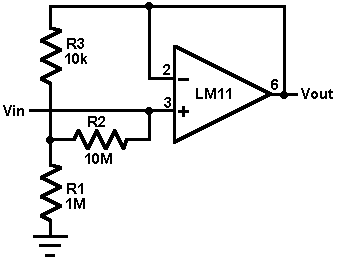# Voltage follower with 1G ohm input resistance Electronic Schematics > Test and measurement > Voltage follower with 1G ohm input resistanceThis circuit uses an LM11 to form a voltage follower with 1G ohm input resistance built using standard resistor values. With the input disconnected, the input offset voltage is multiplied by the same factor as R2; but the added error is small because the offset voltage of the LM11 is so low. When the input is connected to a source less than 1G ohm, this error is reduced. For an ac-coupled input a second 10M resistor could be connected in series with the inverting input to virtually eliminate bias current error; bypassing it would give minimal noise.

Title: Voltage follower with 1G ohm input resistance
electronic circuit
Source: National Semiconductor
Published on: 2005-02-10
Reads: 501
Print version:## Other electronic circuits and schematics from Test and measurement

Electronic circuits > Test and measurement > Voltage follower with 1G ohm input resistance